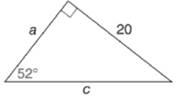Chapter 11.3, Problem 18E### Elementary Geometry for College St...

6th Edition
Daniel C. Alexander + 1 other
ISBN: 9781285195698

#### Solutions

Chapter
Section### Elementary Geometry for College St...

6th Edition
Daniel C. Alexander + 1 other
ISBN: 9781285195698
Textbook Problem
1 views

# In Exercises 15 to 20, use the sine, cosine, or tangent ratio to find the lengths of the indicated sides to the nearest tenth of a unit.To determine

To find:

The lengths of the indicated sides to the nearest tenth of a unit using the sine, cosine or tangent ratio.

Explanation

Given:

The triangle with the indicated sides is shown below.

Definition used:

The trigonometric ratios:

Calculation:

Let α=52.

From the figure we see that opposite=20,adjacent=a,hypotenuse=c.

We need to find the values of a, c.

We have sinα=oppositehypotenuse

sin52=20c

tan52=20a

Let us use Ti-83 calculator to find the value of sin52,tan52.

Press ON in Ti-83 calculator.

Since we need to find the value of degree, we need to change the mode to degrees.

Press MODE button and use arrow keys to select the “Degree” mode.

Let us find the values, correct to four decimal places.

Since we need the values of sin52,tan52 correct to four decimal places, in MODE, using arrow keys choose the value of “Float” as 4

### Still sussing out bartleby?

Check out a sample textbook solution.

See a sample solution

#### The Solution to Your Study Problems

Bartleby provides explanations to thousands of textbook problems written by our experts, many with advanced degrees!

Get Started

#### 10003/2

Applied Calculus for the Managerial, Life, and Social Sciences: A Brief Approach

#### Evaluate . e + 1

Study Guide for Stewart's Single Variable Calculus: Early Transcendentals, 8th

#### True or False: converges.

Study Guide for Stewart's Multivariable Calculus, 8th